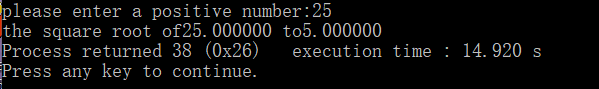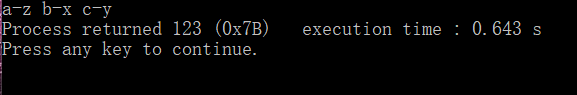# C程序设计 谭浩强 第五章课后习题详解（包含算法分析，运行结果）

## 第五章 循环结构程序设计

### （3）输入m,n 求最大公约数、最小公倍数

# include<stdio.h>
void main()
{
int m,n,c,d,e;
scanf("%d %d",&m,&n);
while(1)
{c=m%n;
if(c==0)
{
d=m/n;
printf("%d是最小公倍数\n",e=d*n);
printf("%d是最大公约数",n);
break;}
else
{m=n;
n=c;}

}}

### （4）输入一行字符，分别统计英文字母、空格、数字、其他字符的个数

 include<stdio.h>
void main ()
{
char c;
int num=0,letter=0,other=0,blank=0;
printf("请输入字符，输入回车结束");
while((c=getchar())!='\n')
{
if(c>='A'&&c<='z')
letter++;
else if(c==' ')
blank++;
else if(c>='0'&&c<='9')//虽然是数字，但是字符进行比较也要加单引号
num++;

else
other++;
}
printf("包含数字%d 包含字母%d 其他%d 包含空格%d",num,letter,other,blank);
}


### （5）S1...Sn,2+22+222+2222+22222(n=5),a是一个数字，n由键盘输入

#### 算法分析：数字的增长是以10的倍数在加2

# include<stdio.h>
void main()
{
int n,i,k=2,s=0;
scanf("%d",&n);
for(i=1;i<=n;i++)
{
s=s+k;
k=k*10+2;
}
printf("%d",s);
}

### （6），1!+2!+3!+...+20!

#### 算法分析：外边的for用来循环1...20，里面的用来计算阶乘

# include <stdio.h>
void main()
{
for(i=1;i<=20;i++)
{for(j=1;j<=i;j++)
printf("%d",sum);
}



### （7）

#### 算法分析：使用三个for循环完成三个累加公式，最后再将结果相加

# include <stdio.h>
int main()
{
int k;
for(k=1;k<=100;k++)
for(k=1;k<=50;k++)
for(i=1;i<=10;i++)
}

### （8）输出所有的水仙花数

# include <stdio.h>
void main()
{
int i=100,a,b,c,d;
for(i;i<1000;i++)
{
a=i/100;   //得到百位
b=i%100/10;//得到十位
c=i%100%10;//得到个位
d=a*a*a+b*b*b+c*c*c;
if(d==i)
printf("%d ",i);
}
}


### （9）找出1000以内的所有完数

#### 算法分析：这道题比较复杂，在输出的时候要从新判断

#include <stdio.h>
void main()
{
int i,j,sum,k;
for(i=1;i<1000;i++)         //1000以内
{
sum=0;                 //sum=0要放在循环体内，不然判断完一个数后不会归零
for(j=1;j<i;j++)       //遍历因子
{if(i%j==0)        //判断是否是完数
sum=sum+j;}
if(sum==i)
{printf("%d its factors are ",sum);
for(j=1;j<i;j++)  //输出因子
{
if(i%j==0)
printf("%d ,",k=j);}
printf("\n");}
}
}

### （10）分数序列的前20项和

#### 算法分析：观察该分数序列，发现既不是等比数列也不是等差数列，那么就从数列中找规律，发现从第二项开始分子是前一项分子分母的和，分母是前一项的分子。设计算法（注意除数应尽可能地为带小数点的实数）

int main()
{
int i,k,t;
double j,sum;

i=1;j=2;
sum=0;
for(k=1;k<=20;k++)
{
sum=sum+j/i;
t=j;j=i+j;i=t;
}
printf("%f",sum);
return 0;
}

### （11）100米自由落下，每次反跳原先的一半，求第十次落地，共经多少米，第十次反弹多高

#### 算法分析：这道题的易错点在于要从第二次开始循环，我一开始也犯了错，原因在于第一次落地的距离是不需要叠加反弹距离的。第一次落地共100米，第一次反弹50米；第二次落地共两百米，第二次反弹25米，一直到第10次，仔细揣摩题意。

# include <stdio.h>
void main()
{
int i;
float l=100;
float d=100/2;   //l用来计算共经多少米，d用来计算第反弹高度
for(i=2;i<=2;i++)//第二次才开始加反弹距离，所以要从第二次开始循环
{
l=l+2*d;//全程计算反弹高度，如果不计算的话l=l+d
d=d/2 ;
}
printf("第十次反弹%f米",d);
printf("第十次落地后共经过%f米",l);
}

### （12）猴子吃桃，每次一半再多吃一个，十天后只剩一个

#### 算法分析：这道题主要要搞明白前一天的桃子树与前一天的关系。

# include <stdio.h>
void main()
{
int peach=1;//第一天剩1个
int i;//i天
for(i=2;i<=10;i++)
{
peach=(peach+1)*2;//前一天的桃子是后一天加一后乘二
}
printf("%d",peach);
}



### （13）迭代法，x等于根号下a，公式Xn+1=1/2(Xn+a/Xn)

#### 下图示例为正确程序

# include <stdio.h>
void main()
{
float a,x0,x1;
scanf("%f",&a);
x0=a/2; //x0的值可以任意选取，输入的值为未知，使用除2得到的数一般比开平方后的值小
x1=(x0+a/x0)/2;//注意：要共同的到x0,x1的值才开始迭代（放入循环体）
do
{
x0=x1;
x1=(x0+a/x0)/2;
}
while(fabs(x1-x0)>=1e-5);//当满足条件绝对值小于10的-5后跳出循环体
printf("the square root of%f to%f",a,x1);
}#### 举一个错误程序（也是我犯的错误）

# include <stdio.h>
void main()
{
float a,x0,x1;
scanf("%f",&a);
x0=a/2; //x0的值可以任意选取，输入的值为未知，使用除2得到的数一般比开平方后的值小
do
{
x1=(x0+a/x0)/2;
x0=x1;

}
while(fabs(x1-x0)>=1e-5);//当满足条件绝对值小于10的-5后跳出循环体
printf("the square root of%f to%f",a,x1);
}


### （14）牛顿迭代法，在1.5附近的根，2x^3-4x^2+3x-6=0

#### 算法分析：知道牛顿迭代公式，x1=x0-f(x0)/f'(x0)，迭代限制在两次求出x的差的绝对值小于10的负五，这块应该是可以通用的。

# include <stdio.h>
# include <math.h>
float main()
{
float  x1,x0,f1,f0;
x1=1.5;
do
{
x0=x1;
f0=((2*x0-4)*x0+3)*x0-6;
f1=(6*x0-8)*x0+3;
x1=x0-f0/f1;
}
while(fabs(x0-x1)>=1e-5);
printf("%f",x1);
}


### （15）二分法，（-10，10）之间的根，2x^3-4x^2+3x-6=0

# include<stdio.h>
# include<math.h>
void main()
{
float x0,x1,x2,f1,f2,f0;
x1=-10;
x2=10;      //将范围定在（-10,10)
do{
f1=((2*x1-4)*x1+3)*x1-6;
f2=((2*x2-4)*x2+3)*x2-6;
}while(f1*f2>0);//第一步f(a)*f(b)异号根据介值定理有方程的根
do{
x0=(x1+x2)/2;//第二步求区间的中点
f0=((2*x0-4)*x0+3)*x0-6;
if(f0*f1>0)//第三部判断f0与f1是否异号,例同号[m,b],异号[a,m]
{x1=x0;
f1=f0;}
else
{x2=x0;
f2=f0;}
}
while(fabs(f0)>=1e-5);//判断所求根的函数值是否满足要求，通用
printf("%f",x0);//输出合格的根
}


### （16）输出图形

#### 算法分析：一个图形，但分为两大步做，两个for循环，每个for循环中分别有对空格、星号输出的for循环。

void main()
{
int i,j,k;
for(i=0;i<=3;i++)
{
for(j=0;j<=2-i;j++)//这里需要注意j<=2-i
printf(" ");
for(k=0;k<=2*i;k++)
printf("*");
printf("\n");
}

for(i=0;i<=2;i++)
{
for(j=3;j>2-i;j--)
printf(" ");
for(k=0;k<=4-2*i;k++)
printf("*");
printf("\n");
}
}

### （17）乒乓球队，abc，xyz

#### 算法分析：简单来说这道题相当于字符的比较，先确保a,b,c的对手不一样，然后再添加条件，进行输出，初学比较难想出来。

# include <stdio.h>
void main()
{
char a,b,c;       //a是z的对手，c是y的对手，x是b的对手
for(a='x';a<='z';a++)
{for(b='x';b<='z';b++)
if(a!=b)
for(c='x';c<='z';c++)
if(a!=c&&b!=c)       //确保a,b,c的对手都是不一样后
if(a!='x'&&c!='x'&&c!='z')//添加条件
printf("a-%c b-%c c-%c",a,b,c);}
}06-28
01-10454
04-05637
10-03207
05-292万+
11-251746
10-12
06-22
07-16
02-07
02-07
06-305376
05-171476
03-23212
09-11245

### “相关推荐”对你有帮助么？

•非常没帮助
•没帮助
•一般
•有帮助
•非常有帮助被折叠的  条评论 为什么被折叠?到【灌水乐园】发言¥1 ¥2 ¥4 ¥6 ¥10 ¥20获取中扫码支付点击重新获取扫码支付1.余额是钱包充值的虚拟货币，按照1:1的比例进行支付金额的抵扣。
2.余额无法直接购买下载，可以购买VIP、付费专栏及课程。余额充值